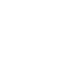# SAT Question of the Day (ACT bonus!): Mar 11, 2014

If you are reading this in an email you received from me, do not click the link to sat.collegeboard.org below. Use the link to my website that is farther down on the email.

http://sat.collegeboard.org/practice/sat-question-of-the-day?questionId=20140311&oq=1 (This link takes you to today’s question. If you use my archive, you will see the question related to my SAT explanation for that date.)

There are lots of students missing this question but that doesn’t mean it is hard!  Only 31% are getting it correct and you can get it right in about 30 seconds if you use my favorite math strategy: “change the abstract to the concrete” (or as I sometimes call it “change the algebra to arithmetic” or “substitute numbers for variables.”  It’s simple to do these problems because when the test writers don’t give you numbers, you get to make them up!

Whenever the test writers give you only variables and not numbers, follow the rules they give you and “change the variables to numbers,” or “substitute the abstract with the concrete,” or “change the algebra to arithmetic.”  They are all the same.  All you need to do is substitute numbers for the unknowns.  Let’s use my strategy for this question.

The answer is E.  Substitute 6 for c and 12 for g.  (Any other numbers will work but be sure to be gentle on yourself and avoid using numbers that require a calculator.)  Now you can see that each car needs two gallons per week.  Therefore, 5 cars would use 10 gallons in one week and 20 gallons in two weeks; that’s your answer, 20 gallons.  Now just plug 6 in for c and 12 in for g into the answers and see which one gives you 20!  The only one that works is E.

Why does this strategy work so easily?  The answer is, “The world of math is a world of patterns.”  For example, you could have been very easy on yourself and said c = 1 and g =2.  Then you would know that in two weeks that one car would use 4 gallons and so 5 cars would use 20.  Now plug in 1 for c and 2 for g into the answers.  Oops!  Both Answers A and E work.  (That is because 1 times 2 (for Answer A) and 2 divided by 1 (for Answer E) are both equal to 2.  (Now you know why I recommend avoiding the use of 1 when you substitute numbers for variables.)  Just change your numbers.  You could use 2 for c and 6 for g.  Each car would use 3 gallons for one week and 6 for two weeks.  Five cars would need 30 gallons.  Answer A gives you 60 and Answer C gives you 30 and you are all done.

I find my strategy faster and more accurate than doing the algebra (even though you could certainly do it that way).  Numbers are easier to manipulate than variables; you’ve been doing it since kindergarten.  You are also less prone to making silly mistakes with numbers.  How hard is it to multiply 10 times 12 and divide by 6 to get 20  in today’s question using my first numbers?  Not very.  Finally, if the numbers require using a calculator (and you shouldn’t pick ones that do), you can use your calculator.

Remember that getting today’s question either right or wrong isn’t important!  You need to use it to learn that when the test writers don’t give you numbers, you get to make stuff up!  There are going to be a lot (approximately 20%) of questions that don’t give you numbers and you should not be intimidated (Pillar VI).  Just change the algebra (variables) to arithmetic (numbers) and you’ll do fine.  For more practice, use the table on page 21 in my book Demystifying the SAT & ACT.

I wonder if the ACT folks have something new for us this morning.

Here’s an easy question for which you can use the substitution strategy.  In this case, they don’t give you numbers but they sure give you abstract values since the questions are about square roots of non-perfect squares.  But wait!!  The numbers are really close to perfect squares: 50 is close to 49 (with a square root of 7).  And 80 is close to 81 (with a square root of 9).  So, 7 times 9 is 63.  Game over because the puzzle is solved.

You could also have used your calculator to determine the square roots of 50 and 80; then you could have multiplied those two amounts.  No problem.  But I bet you could have answered the question more quickly by using approximations and multiplying in your head.  The test writers even made it easy because none of the other answers are even close to 63 which would help eliminate any silly mistakes.

Both of these questions help remind you that the challenge of the math tests isn’t simply doing math.  That can take a lot of time.  These questions show you how important it is to be able to think about math and recognize the patterns you will see on the tests.

I hope you have a great day.  Get that assignment out of the way that you’ve been putting off.  That would make it a wonderful day.

Bob Alexander, the “SAT and ACT Wizard”